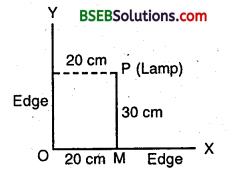# HSSlive: Plus One & Plus Two Notes & Solutions for Kerala State Board

## BSEB Class 9 Maths Chapter 3 Coordinate Geometry Ex 3.1 Textbook Solutions PDF: Download Bihar Board STD 9th Maths Chapter 3 Coordinate Geometry Ex 3.1 Book AnswersBSEB Class 9 Maths Chapter 3 Coordinate Geometry Ex 3.1 Textbook Solutions PDF: Download Bihar Board STD 9th Maths Chapter 3 Coordinate Geometry Ex 3.1 Book Answers

BSEB Class 9th Maths Chapter 3 Coordinate Geometry Ex 3.1 Textbooks Solutions and answers for students are now available in pdf format. Bihar Board Class 9th Maths Chapter 3 Coordinate Geometry Ex 3.1 Book answers and solutions are one of the most important study materials for any student. The Bihar Board Class 9th Maths Chapter 3 Coordinate Geometry Ex 3.1 books are published by the Bihar Board Publishers. These Bihar Board Class 9th Maths Chapter 3 Coordinate Geometry Ex 3.1 textbooks are prepared by a group of expert faculty members. Students can download these BSEB STD 9th Maths Chapter 3 Coordinate Geometry Ex 3.1 book solutions pdf online from this page.

## Bihar Board Class 9th Maths Chapter 3 Coordinate Geometry Ex 3.1 Books Solutions

 Board BSEB Materials Textbook Solutions/Guide Format DOC/PDF Class 9th Subject Maths Chapter 3 Coordinate Geometry Ex 3.1 Chapters All Provider Hsslive

## How to download Bihar Board Class 9th Maths Chapter 3 Coordinate Geometry Ex 3.1 Textbook Solutions Answers PDF Online?

2. Click on the Bihar Board Class 9th Maths Chapter 3 Coordinate Geometry Ex 3.1 Answers.
3. Look for your Bihar Board STD 9th Maths Chapter 3 Coordinate Geometry Ex 3.1 Textbooks PDF.
4. Now download or read the Bihar Board Class 9th Maths Chapter 3 Coordinate Geometry Ex 3.1 Textbook Solutions for PDF Free.

## BSEB Class 9th Maths Chapter 3 Coordinate Geometry Ex 3.1 Textbooks Solutions with Answer PDF Download

Find below the list of all BSEB Class 9th Maths Chapter 3 Coordinate Geometry Ex 3.1 Textbook Solutions for PDF’s for you to download and prepare for the upcoming exams:

## BSEB Bihar Board Class 9th Maths Solutions Chapter 3 Coordinate Geometry Ex 3.1

Question 1.
How will you describe, the position of a table lamp on your study table to another person?
Solution:
Consider the lamp as a point P and table as a plane. Choose any two perpendicular edges of the table, say OX and OY. Measure the distance of the lamp i.e., P from the longer edge OX, let it be 30 cm. Again, measure the distance of the lamp P from the shorter edge OY, let it be 20 cm.Thus, the position of the lamp P referred to the edges OX and OY is (20, 30).

Question 2.
(Street Plan) : A city has two main roads which cross each other at the centre of the’ city. These two roads are along the North-South direction and East- West direction. All-other streets of the city run parallel to these roads and are 200 m apart. There are about 5 streets in each direction. Using 1 cm = 200 m, draw a model of the city on your notebook. Represent the roads/streets by single lines.
There are many cross-streets in your model. A particular cross-street is made by two streets, one running in the North-South direction and another in the East-West direction. Each cross street is referred to in the following manner: If the 2nd street running in the North-South direction and 5th in the East-West direction meet at some crossing, then we will call this cross-street (2, 5). Using this convention, find :
(i) how many cross-streets can be referred to as (4,3)
(ii) how many cross-streets can be referred to as (3, 4)
Solution:
Street plan is as shown in the figure :(i) There is only one cross street, which can be referred as (4, 3).
(ii) There is only one cross street, which can be referred as (3, 4).

## Bihar Board Class 9th Maths Chapter 3 Coordinate Geometry Ex 3.1 Textbooks for Exam Preparations

Bihar Board Class 9th Maths Chapter 3 Coordinate Geometry Ex 3.1 Textbook Solutions can be of great help in your Bihar Board Class 9th Maths Chapter 3 Coordinate Geometry Ex 3.1 exam preparation. The BSEB STD 9th Maths Chapter 3 Coordinate Geometry Ex 3.1 Textbooks study material, used with the English medium textbooks, can help you complete the entire Class 9th Maths Chapter 3 Coordinate Geometry Ex 3.1 Books State Board syllabus with maximum efficiency.

## FAQs Regarding Bihar Board Class 9th Maths Chapter 3 Coordinate Geometry Ex 3.1 Textbook Solutions

#### Can we get a Bihar Board Book PDF for all Classes?

Yes you can get Bihar Board Text Book PDF for all classes using the links provided in the above article.

## Important Terms

Bihar Board Class 9th Maths Chapter 3 Coordinate Geometry Ex 3.1, BSEB Class 9th Maths Chapter 3 Coordinate Geometry Ex 3.1 Textbooks, Bihar Board Class 9th Maths Chapter 3 Coordinate Geometry Ex 3.1, Bihar Board Class 9th Maths Chapter 3 Coordinate Geometry Ex 3.1 Textbook solutions, BSEB Class 9th Maths Chapter 3 Coordinate Geometry Ex 3.1 Textbooks Solutions, Bihar Board STD 9th Maths Chapter 3 Coordinate Geometry Ex 3.1, BSEB STD 9th Maths Chapter 3 Coordinate Geometry Ex 3.1 Textbooks, Bihar Board STD 9th Maths Chapter 3 Coordinate Geometry Ex 3.1, Bihar Board STD 9th Maths Chapter 3 Coordinate Geometry Ex 3.1 Textbook solutions, BSEB STD 9th Maths Chapter 3 Coordinate Geometry Ex 3.1 Textbooks Solutions,
Share: